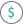#EFFECT (PQL - xl)

This function calculates the effective annual interest rate for a given nominal annual interest rate and the number of compounding periods per year

• Library: PQL \ Spreadsheet \ Financial
• Compatibility: Any content (regardless of data source) in the Tabulate spreadsheet module

#### Syntax

EFFECT(nominal rate, npery)

##### Function Arguments
 Name Description Type Optional nominal rate Nominal interest rate Number npery Number of compounding periods; if not an integer, the number is truncated Number